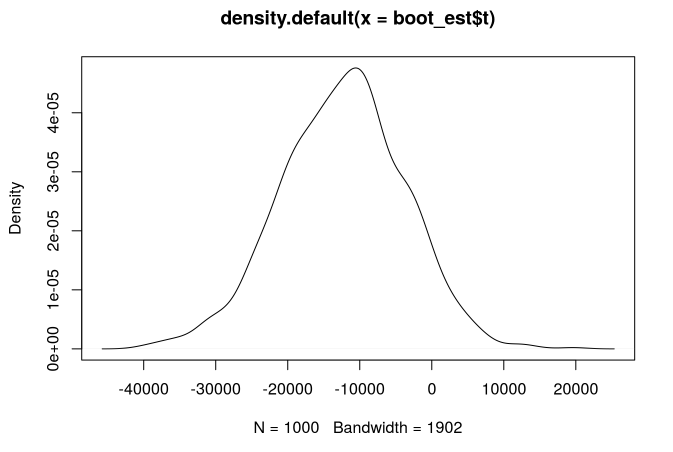Have a question about one of the blog posts? Chat here with me.

# Bootstrapping standard errors for difference-in-differences estimation with R

R

I’m currently working on a paper (with my colleague Vincent Vergnat who is also a Phd candidate at BETA) where I want to estimate the causal impact of the birth of a child on hourly and daily wages as well as yearly worked hours. For this we are using non-parametric difference-in-differences (henceforth DiD) and thus have to bootstrap the standard errors. In this post, I show how this is possible using the function boot.

For this we are going to replicate the example from Wooldridge’s Econometric Analysis of Cross Section and Panel Data and more specifically the example on page 415. You can download the data for R here. The question we are going to try to answer is how much does the price of housing decrease due to the presence of an incinerator in the neighborhood?

First put the data in a folder and set the correct working directory and load the boot library.

library(boot)
setwd("/home/path/to/data/kiel data/")
load("kielmc.RData")

Now you need to write a function that takes the data as an argument, as well as an indices argument. This argument is used by the boot function to select samples. This function should return the statistic you’re interested in, in our case, the DiD estimate.

run_DiD <- function(my_data, indices){
d <- my_data[indices,]
return(
mean(d$rprice[d$year==1981 & d$nearinc==1]) - mean(d$rprice[d$year==1981 & d$nearinc==0]) -
(mean(d$rprice[d$year==1978 & d$nearinc==1]) - mean(d$rprice[d$year==1978 & d$nearinc==0]))
)
}

You’re almost done! To bootstrap your DiD estimate you just need to use the boot function. If you have cpu with multiple cores (which you should, single core machines are quite outdated by now) you can even parallelize the bootstrapping.

boot_est <- boot(data, run_DiD, R=1000, parallel="multicore", ncpus = 2)

Now you should just take a look at your estimates:

boot_est

ORDINARY NONPARAMETRIC BOOTSTRAP

Call:
boot(data = data, statistic = run_DiD, R = 1000, parallel = "multicore",
ncpus = 2)

Bootstrap Statistics :
original    bias    std. error
t1* -11863.9 -553.3393    8580.435

These results are very similar to the ones in the book, only the standard error is higher.

You can get confidence intervals like this:

quantile(boot_est$t, c(0.025, 0.975)) ## 2.5% 97.5% ## -30186.397 3456.133 or a t-statistic: boot_est$t0/sd(boot_est$t) ##  -1.382669 Or the density of the replications: plot(density(boot_est$t))Just as in the book, we find that the DiD estimate is not significant to the 5% level.